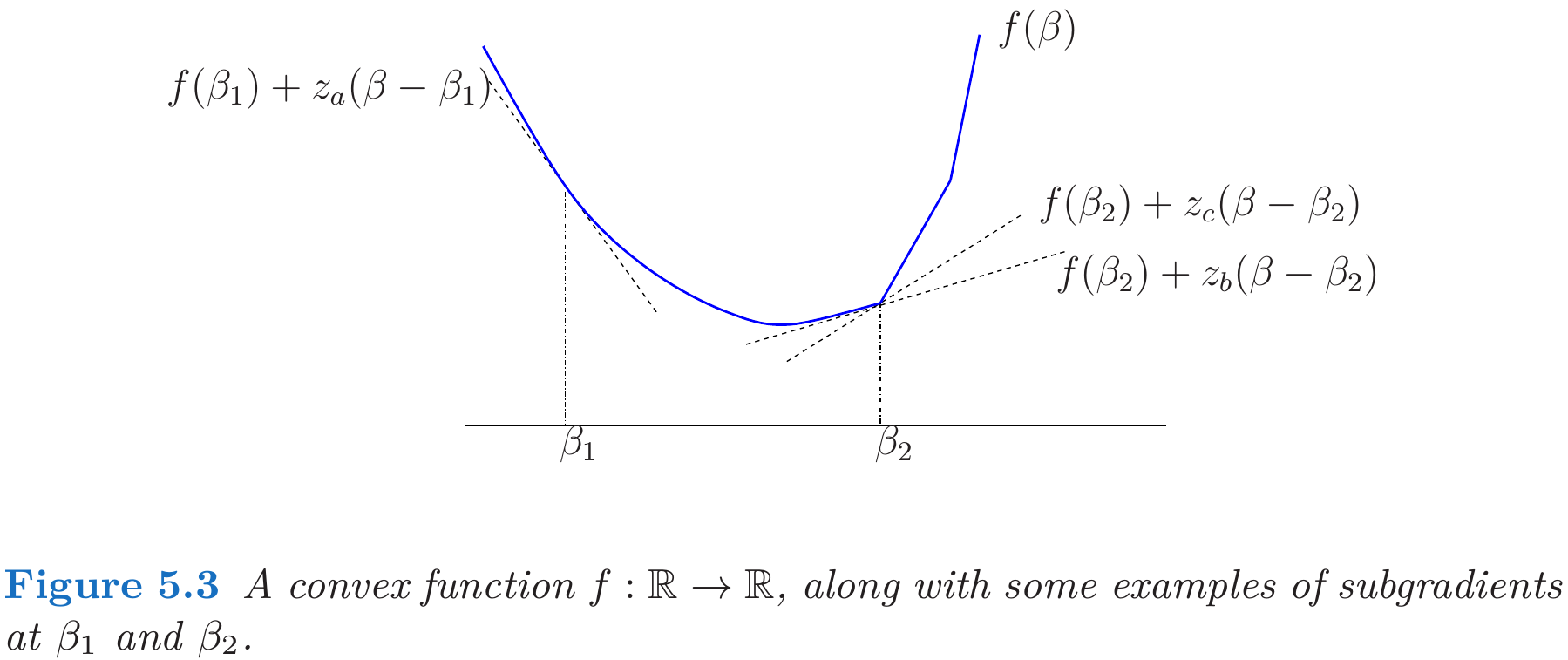# WeiYa's Work Yard

##### Posted on Apr 08, 2019

This post is mainly based on Hastie et al. (2015), and incorporated with some materials from Watson (1992).

Consider the convex optimization problem

$\min_{\beta\in\bbR^p}\;f(\beta)\qquad \text{such that }g_j(\beta)\le 0\text{ for }j=1,\ldots,m\,,$

where $g_j$ are convex functions.

The Lagrangian $L$ is defined as

$L(\beta;\lambda) = f(\beta) + \sum_{j=1}^m\lambda_jg_j(\beta)\,,$

where the nonnegative weights $\lambda\ge 0$ are known as Lagrange multipliers.

For differentiable problems, the famous KKT (Karush-Kuhn-Tucker) conditions relate the optimal Lagrange multiplier vector $\lambda^*\ge 0$, also known as the dual vector, to the optimal primal vector $\beta^*\in\bbR^p$:

• Primal feasibility: $g_j(\beta^*)\le 0$ for all $j=1,\ldots,m$
• Complementary slackness: $\lambda_j^*g_j(\beta^*)=0$ for all $j=1,\ldots,m$
• Lagrangian conditions: $0=\nabla_\beta L(\beta^*;\lambda^*) = \nabla f(\beta^*)+\sum_{j=1}^m\lambda_j^*\nabla g_j(\beta^*)$.

For nondifferentiable functions, there is a natural generalization of the notion of gradient that allows for a more general optimality theory.

Given a convex function $f:\bbR^p\rightarrow \bbR$, a vector $z\in\bbR^p$ is said to be a subgradient of $f$ at $\beta$ if $$f(\beta') \ge f(\beta) + \langle z,\beta'-\beta\rangle\quad\text{for all }\beta'\in \bbR^p\,.$$ The set of all subgradients of $f$ at $\beta$ is called the subdifferential, denoted by $\partial f(\beta)$.Under mild conditions, the generalized KKT theory can still be applied using the modified condition

$0\in \partial f(\beta^*) + \sum_{j=1}^m \lambda_j^*\partial g_j(\beta^*)\,.$

The remaining parts will give some examples of subgradients.

The necessary and sufficient conditions for a solution to

$\min_{\beta\in\bbR^p} \left\{\frac{1}{2N}\Vert \y-\X\beta\Vert_2^2+\lambda \Vert\beta\Vert_1\right\}\quad \lambda >0$

take the form

$-\frac 1N\langle \x_j,\y-\X\beta\rangle + \lambda s_j=0,\;j=1,\ldots,p\,.$

Here each $s_j$ is an unknown quantity equal to $\sign(\beta_j)$ if $\beta_j\neq 0$ and some value lying in $[-1,+1]$ otherwise, that is, it is a subgradient for the absolute value function $f(\beta)=\vert \beta\vert$,

$\partial f(\beta) = \begin{cases} \{+1\} & \text{if }\beta > 0\\ \{-1\} & \text{if }\beta < 0\\ [-1,+1]& \text{if }\beta = 0 \end{cases}$

Given a matrix $\Theta\in \bbR^{m\times n}$ (assume $m\le n$), by SVD decomposition,

$\Theta=\sum_{j=1}^m\sigma_ju_jv_j^T\,.$

The nuclear norm is the sum of the singular values,

$\Vert \Theta\Vert_*=\sum_{j=1}^m \sigma_j(\Theta)\,.$

The subdifferential $\partial \Vert\Theta\Vert_*$ of the nuclear norm at $\Theta$ consists of all matrices of the form $\Z = \sum_{j=1}^mz_ju_jv_j^T$, where each for $j=1,\ldots,m$, the scalar $z_j\in \sign(\sigma_j(\Theta))$.

Note that we need to verify that

$\Vert \Theta'\Vert_* \ge \Vert \Theta\Vert_* + \tr[\Z^T(\Theta'-\Theta)]$

for all $\Theta’\in\bbR^{m\times n}$. The inner product for matrix $\A$ and $\B$ is defined by $\langle \A, \B\rangle=\tr(\A^H\B)$

For simplicity, consider the case that all singular values are larger than 0, then

\begin{align*} \Vert \Theta\Vert_* + \tr[\Z^T(\Theta'-\Theta)] &= \sum_{j=1}^m \sigma_j + \tr[\V\U^T(\Theta'-\U\D\V^T)] \\ &= \tr[\V\U^T\Theta']\\ &= \tr[\V\U^T\U_*\D_*\V^T_*]\\ &= \sum_{j=1}^m\sigma_j' = \Vert\Theta'\Vert_*\,, \end{align*}

since $\V\U^T\U_*$ can be viewed as the new left singular vectors.

## Subgradient for Orthogonally Invariant Norms

As commented by @Lepidopterist, Watson (1992) completely and thoroughly discussed the derivation of subgradient of (nuclear) matrix norms.

Generally, the subdifferential (or set of subgradients) of $\Vert A\Vert$ is defined by

$\partial \Vert A\Vert =\left\{G:\bbR^{m\times n}: \Vert B\Vert\ge \Vert A\Vert +\tr[G^T(B-A)], \text{for all }B\in\bbR^{m\times n}\right\}\,.$

It is readily established that $G\in \partial \Vert A\Vert$ is equivalent to the statements

1. $\Vert A\Vert = \tr(G^TA)$
2. $\Vert G\Vert^*\le 1$

where

$\Vert G\Vert^* = \max_{\Vert B\vert \le 1}\tr[B^TG]\,,$

and $\Vert \cdot\Vert^*$ is the polar or dual norm to $\Vert \cdot \Vert$.

The class of Orthogonally Invariant Norms consists of norms such that

$\Vert UVA\Vert =\Vert A\Vert$

for any orthogonal matrices $U$ and $V$ of orders $m$ and $n$ respectively. All such norms can be defined by

$\Vert A\Vert = \phi(\bsigma)\,,$

where $\bsigma = (\sigma_1,\ldots,\sigma_n)^T$ is the singular values of $A$, and $\phi$ is a symmetric gauge function. For nuclear norm, $\phi(\bsigma_n) = \sum_{j=1}^n\sigma_j$.

$$\partial \Vert A\Vert = \mathrm{conv}\{UDV^T, A=U\Sigma V^T,\bfd\in \partial \phi(\bsigma)\}\,,$$ where $\mathrm{conv}\{\}$ signifies the convex hull of a set (the smallest convex set that contains the set). Here $U$ and $V$ are orthogonal matrices, while $D$ is $m\times n$ matrix except down the main diagonal.

I think it does not make much differences for different forms of SVD decomposition.